Example Questions

Example Question #4 : How To Find Percentage

A high school student spends a total of 90 minutes per day studying for the ACT, Monday through Friday, then 2 hours a day on the weekend.  What percent of their week is spent studying for the ACT?

6.25%

7.125%

6.8%

5.5%

8%

6.8%

Explanation:

The number of minutes in a week is: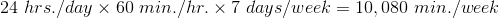The number of minutes spent studying is: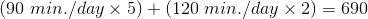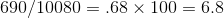6.8%

Example Question #1231 : Act Math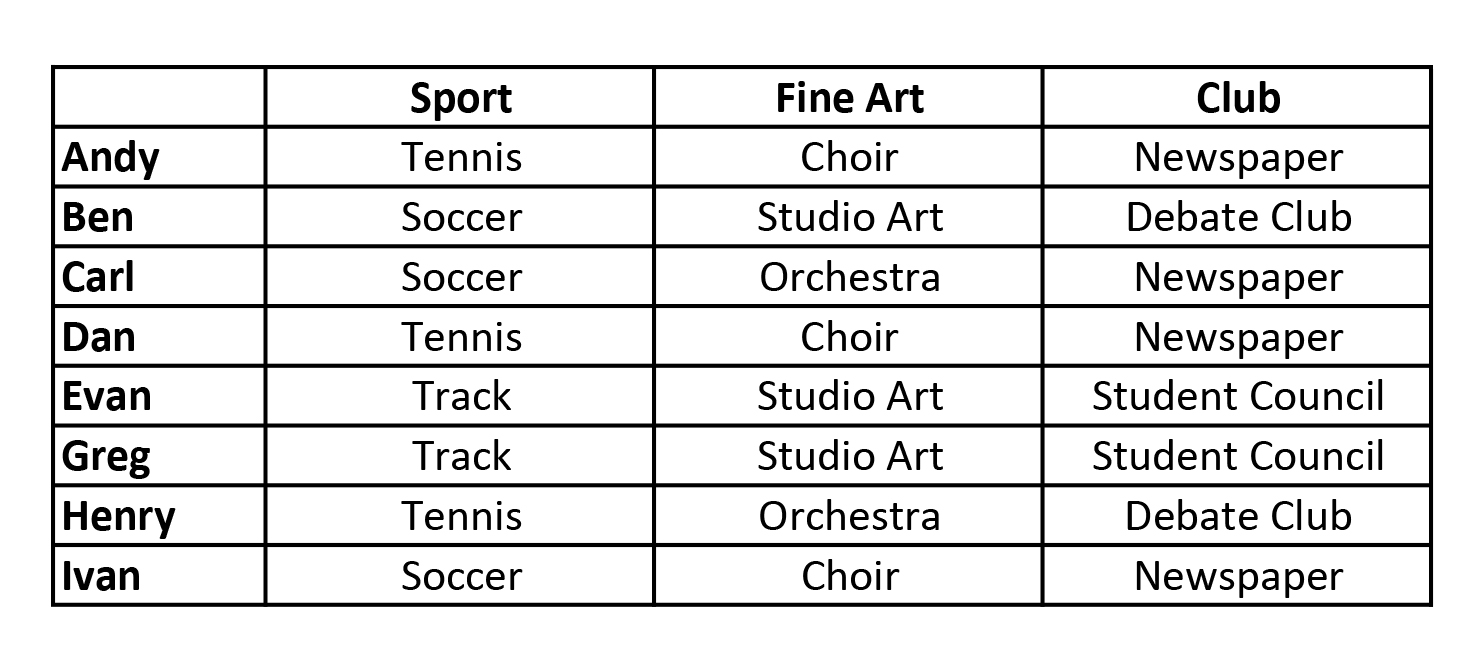Above is a chart showing the students and the different activities they are involved in. What percentage of the students are on the soccer team? Round to the nearest tenth of a percent.

32.5%

40.0%

25.0%

37.5%

30.0%

37.5%

Explanation:

Percentage = Students on Soccer Team / Total Number of Students

= 3/8 = .375 = 37.5%

Example Question #1232 : Act MathWhat percentage of the students are involved in both newspaper and soccer?

15%

25%

20%

30%

35%

25%

Explanation:

There are three students involved in soccer.  Read across the chart and two of them are also involved in newspaper.

Percentage = 2/Total Number of Students

= 2/8 = .25 = 25%

Example Question #1233 : Act Math

The average person spends 30% of their life sleeping.  Assuming the average person lives till the age of 93, how many years will they have spent asleep? Round to the nearest whole number

31 years

9 years

28 years

19 years

30 years

28 years

Explanation:

This problem requires simple arithmetic, 30% of 93 can be obtained by multiply 93 X .30 = 27.9 Rounding yields 28 years.

Example Question #1231 : Act Math

What is 9% of 8,100?

729

459

2,700

900

836

729

Explanation:

8,100 * 0.09 = 729

Example Question #71 : Percentage

76 eleventh-grade students turned in term papers on the United States Constitution. 3 students failed, 26 students recieved C's, 31 students recieved B's. The remaining students earned A's on their papers.

What percentage of students earned A's on their paper? (Round to the nearest percent.)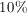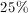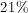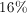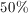Explanation:

Subtract 3, 26, and 31 from 76 to figure out how many students got A's (16).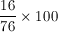Example Question #14 : Other Percentage

What is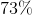of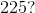Explanation:

To find the percentage of a number, convert the percentage into a decimal by diving the percent by 100.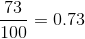,

then multiply that decimal by the number.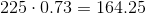Example Question #71 : Percentageis what percent of? Round to the nearest hundredth.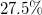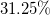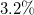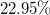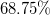Explanation:

For percentage problems, the easiest way to start is by remembering that the word "of" is best translated as a multiplication, while the word "is" is best translated by an equals sign. Thus, for the information provided, we can write the equation: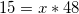Remember, though, thatwill represent a percentage in a decimal form and will need to be translated. (There are other ways of doing this, but most students remember to do this translation at the end naturally.)

Solving for, you get: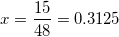This is.

Example Question #16 : Other Percentage

What is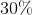of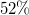of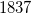?Explanation:

For percentage problems, the easiest way to start is by remembering that the word "of" is best translated as a multiplication, while the word "is" is best translated by an equals sign. Thus, for the information provided, we can write the equation: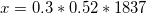Solving for, you get: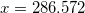Alternatively you could first calculateof, which is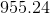, and then calculateof that, and you would arrive at the same answer.

Example Question #17 : Other Percentage

A computer originally sold for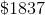dollars. Its price was reduced byand then again by. What was the final sale price for the computer? Round to the nearest cent.Explanation:

The most common way to do a problem like this is to begin by applying the first discount, calculating the amount to be removed from the original price. This is done by multiplying the original price by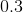. This gives you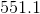. This is then subtracted fromto give you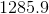. Once again, this new number is multiplied, now by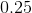. This gives you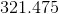. When subtracted from, this gives you a final price of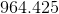, or the rounded value of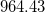.

A simpler way to do this is to realize that the first price reduction makes the new price to be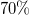of the original. The second will then make the price to be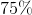of the intermediary price. Thus, you could easily compute: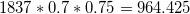All ACT Math Resources